1603675263

# MergeSort Sorting Algorithm in Hindi

Merge Sort Tutorial in Hindi: In this video, we will see how to use merge sort to sort an array of numbers. We will see how to use the merging procedure to merge two sorted arrays into one and how to perform this procedure recursively to sort the entire array!

#algorithm #programming #developer

## Buddha Community1603767600

## Search Algorithms

Today, let us touch base on some fundamental concepts like search algorithms.

In simple terms, **searching **is a process of looking up a particular data record in the database or in the collection of items. A search typically answers as true or false whether the particular data in which we are referring is found or not and perform the next action accordingly.

Commonly used algorithms for search are:

• Linear search
• Binary search
• Interpolation search

Let us understand them in detail with some example

## Linear Search Algorithm

Linear Search Algorithm is the simplest and easiest form of the search algorithm. In this algorithm, a sequential search is made over all the items one by one to search for the targeted item. Each item is checked in sequence until the match is found. If the match is found, the searched item is returned otherwise the search continues till the end.

To make it easy to understand, let us see understand linear search using a flow diagram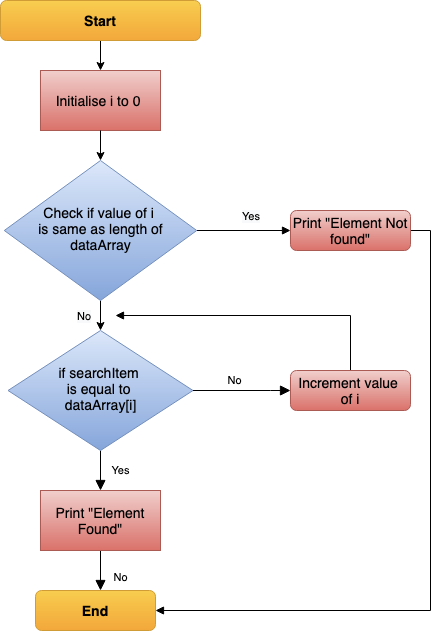Linear Search — Data Flow

### Points to note:

• Does not need sorted array list
• Performs equality comparisons
• The time complexity is O(n)
• Time taken to search elements keeps increasing as the number of elements is increased.

## Binary Search Algorithm

In _Binary search algorithm, _begins with an interval covering the whole array and diving it in half. If the value of the search key is less than the item in the middle of the interval, narrow the interval to the lower half. Otherwise narrow it to the upper half. Repeatedly check until the value is found or the interval is empty.

To make it easy to understand, let us see understand binary search using flow diagram and example as below.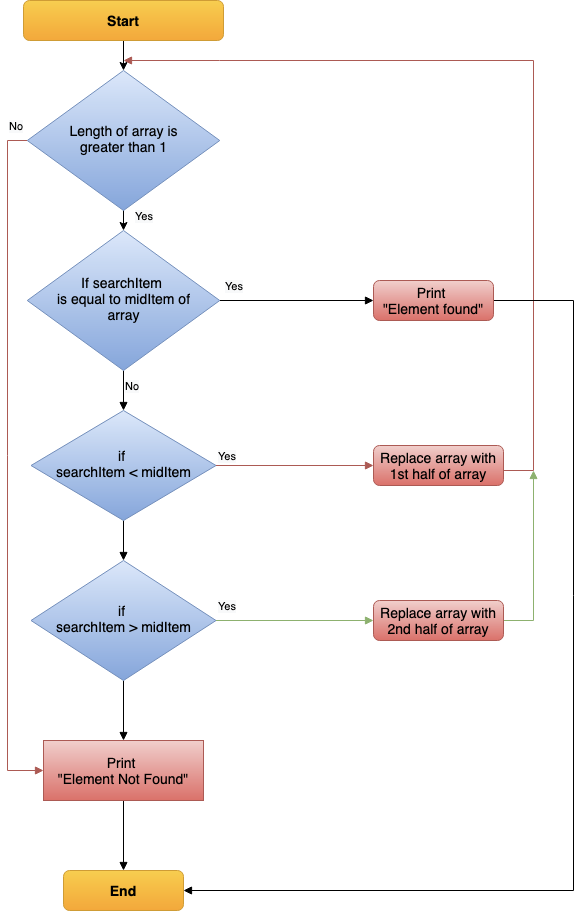Binary Search — Data Flow

### Points to note:

• The array needs to be sorted
• Performs ordering comparisons
• Time complexity to O(log n).
• Search is done to either half of the given list, thus cut down your search to half time

#sorting-algorithms #algorithms #data-structures #search-and-sort #searching-algorithm

1599645721

## Bubble Sort Algorithm Demystified

As Bubble Sort represents the basic foundations of sorting, we’ve tried to demystify this sorting algorithm.

#bubble-sort #sorting #sorting-algorithm #array #practice1592698920

## Selection Sort Algorithm Visually Explained

The Selection Sort algorithm sorts an array by looking for the smallest item and moving it to the front of the list. That’s really all you have to know. Let’s jump into an example.
Selection Sort starts by setting the first value as the minimum value.
It then starts comparing it with each value in the array. If a lower value is found, the minimum value is updated, and the traversal continues until the end of the array is reached. During the first comparison, the value at index 1 is compared to the minimum value.

#programming #algorithms #computer-science #sorting-algorithms #selection-sort1593542940

## Heap Sort Explained

As you may know, heapsort is also one of the most asked sorting algorithms besides mergesort and quicksort and today we’re going to talk about it and I’ll try my best to explain it in much simpler terms as for a beginner it can be quite complicated more so if one has less knowledge about heaps. But fear not I’ve got your back. So now let’s get into it.

Before moving on to heap sort you need to know about the following terminologies to properly understand the working of heapsort.

1. Heaps
2. Heap Property
3. Heapify

So let’s begin by explaining the terms stated above

# 1. Heaps

Heap data structure is a**_ complete binary tree that follows the heapify property_**. So what’s a complete binary tee you might ask?. A complete binary tree follows these conditions.

• All nodes in every level of the tree are filled except the leaf nodes.
• The nodes are as far left as possible.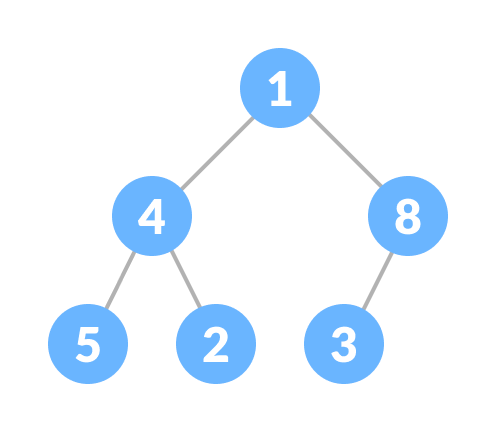Complete Binary Tree

The heap is shown below following the heap property and this is called Max heap. It has the same root and the child nodes but there is a slight difference. The Complete Binay Tree doesn’t care whether it’s root node is smaller or larger but it is of grave importance in case of the heap data structure.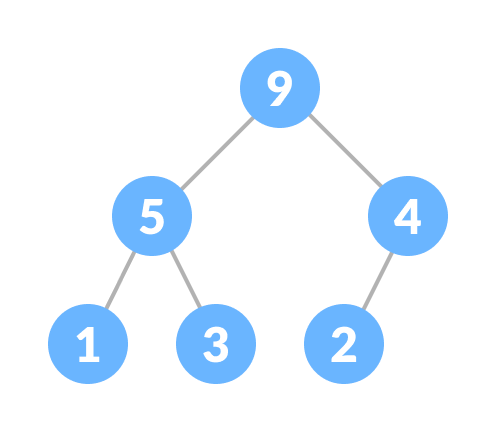Heap

# 2. Heap Property

While creating a heap it is essential to follow the heap property which states as follows:

• The heap where the parent node is always greater than the child nodes and provided that the root node is largest is called a Max-heap.Max Heap

• The heap in which the child node is always greater than it’s child nodes and the root node is the smallest node is called a Min-heap.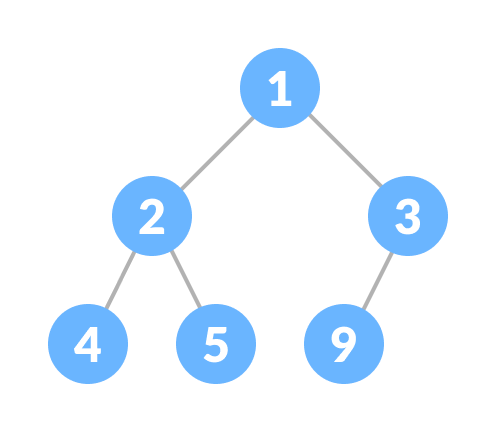Min heap

# 3. Heapify

Heapify is the** process of creating a heap data structure from a binary tree. **Now Heapify is the most important operation on a heap data structure as it helps to maintain the structure of the heap after several operations have been performed on the heap like(insertion, deletion). We will be using this operation a lot and it will come in handy while designing heap sort.

working of heapify is shown below

1. Select an input array.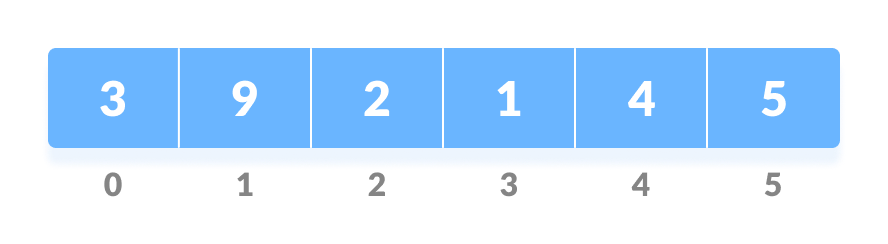Input array

2. Convert this array into a complete binary tree.

Complete binary tree created from the array

3. Create a heap from the binary tree.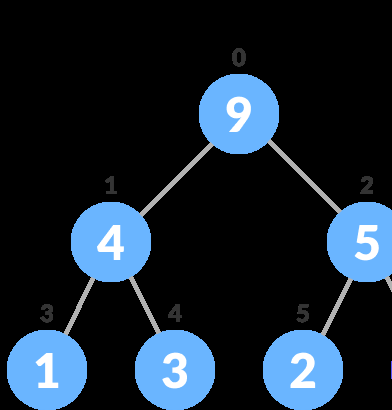After Heapify a max heap is created

Now that we’ve learned about all the important terms let’s start to create heap sort algorithm.

In a heap sort program, we’ll be given an unsorted array and we have to sort it. But heapsort works somewhat different than other sorting algorithms. By different, means the arrangement of each element after operations are performed. In this, the larger elements are sorted first in the last place then followed by the smaller elements, and at last, when there’s one element left that element would be the smallest and the last to go in it’s sorted place.

#sorting #sorting-algorithms #heap #heapsort #sort1596427980

## Explanation of Quick Sort

In this article, I will be talking about quick sort & all the components that you need to know.

Quicksort is nothing but another algorithm to sort a list of items in ascending or descending order. To understand quick sort, we need to understand the following items:

• pivot
• how partition works
• recursion within quick sort & the base case

Pivot is the element that will be used to divide the list of values less than pivot to the left(or the right, if we are sorting it the other direction) and values greater than the pivot to the right of the pivot.

Let’s say we have the following:

``````/* Example array: 4 1 5 2 10 8 6 3 */

const array = [4,1,5,2,10,8,6,3];

//the first element,4, or the last element,3, is commonly used as a pivot in quick sort.

//after partition:
[?,?,3,?,?,?,?]
view raw
pivot.js hosted with ❤ by GitHub
``````

If 3 is chosen as the pivot, it will always land at where it should be left after a partition. In this case, that would be the 2nd index of the array.

To further demonstrate how that would work, view the following illustration: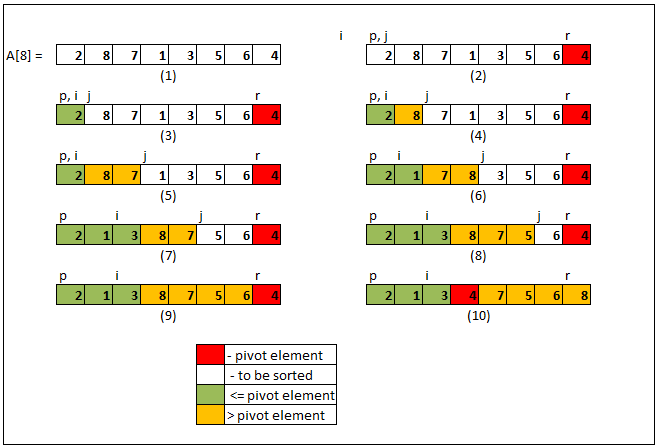On the illustration above, we have i, j, and the pivot. 4 is used as the pivot. i, initially, is set to -1. This indicates the number of elements we have that are less than the pivot. j, on the other hand, is used as an index to traverse through every element.

In partition, for every element j, if the pivot is great than arr[j], increment i and swap arr[i] and arr[j]. This will help put whatever element less than pivot on the left side of the array. J will increment regardless.

After the whole array is done, i equates to the number of elements that are less the pivot. Increment i by 1 and swap arr[i] with the pivot. Now the pivot is truly the dividing point between elements greater than it to the right and elements lesser than it to the left.

Afterward, quicksort will be called on the subarray to the left of the pivot and also to the right of the pivot. This process will repeat until there are no more subarrays to be sorted.

#sorting-algorithms #es6 #algorithms #javascript #web-development #algorithms